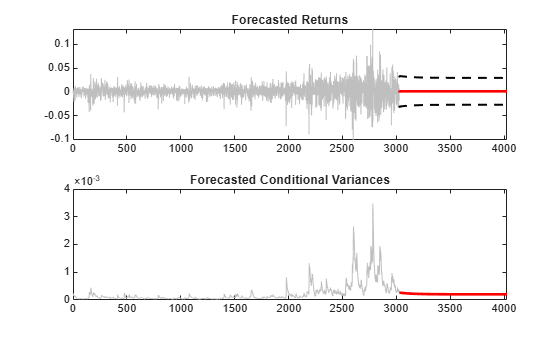# Forecast Conditional Mean and Variance Model

This example shows how to forecast responses and conditional variances from a composite conditional mean and variance model.

### Step 1. Load the data and fit a model.

Load the NASDAQ data included with the toolbox. Fit a conditional mean and variance model to the data.

```load Data_EquityIdx nasdaq = DataTable.NASDAQ; r = price2ret(nasdaq); N = length(r); Mdl = arima('ARLags',1,'Variance',garch(1,1),... 'Distribution','t'); EstMdl = estimate(Mdl,r,'Variance0',{'Constant0',0.001});```
``` ARIMA(1,0,0) Model (t Distribution): Value StandardError TStatistic PValue __________ _____________ __________ __________ Constant 0.00094856 0.00017077 5.5547 2.7812e-08 AR{1} 0.1398 0.019072 7.33 2.3009e-13 DoF 8.4355 1.0404 8.1078 5.1565e-16 GARCH(1,1) Conditional Variance Model (t Distribution): Value StandardError TStatistic PValue __________ _____________ __________ __________ Constant 1.6381e-06 6.1851e-07 2.6485 0.0080856 GARCH{1} 0.89623 0.011251 79.656 0 ARCH{1} 0.095744 0.011012 8.6948 3.4751e-18 DoF 8.4355 1.0404 8.1078 5.1565e-16 ```
`[E0,V0] = infer(EstMdl,r);`

### Step 2. Forecast returns and conditional variances.

Use `forecast` to compute MMSE forecasts of the returns and conditional variances for a 1000-period future horizon. Use the observed returns and inferred residuals and conditional variances as presample data.

```[Y,YMSE,V] = forecast(EstMdl,1000,r,'E0',E0,'V0',V0); upper = Y + 1.96*sqrt(YMSE); lower = Y - 1.96*sqrt(YMSE); figure subplot(2,1,1) plot(r,'Color',[.75,.75,.75]) hold on plot(N+1:N+1000,Y,'r','LineWidth',2) plot(N+1:N+1000,[upper,lower],'k--','LineWidth',1.5) xlim([0,N+1000]) title('Forecasted Returns') hold off subplot(2,1,2) plot(V0,'Color',[.75,.75,.75]) hold on plot(N+1:N+1000,V,'r','LineWidth',2); xlim([0,N+1000]) title('Forecasted Conditional Variances') hold off```The conditional variance forecasts converge to the asymptotic variance of the GARCH conditional variance model. The forecasted returns converge to the estimated model constant (the unconditional mean of the AR conditional mean model).